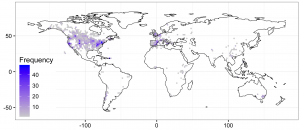## Mapping with ggplot2: stat_binhex maps

Just some notes on the R code used for producing the map on my previous entry about erosion rates across the World.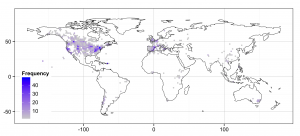Sometimes, when one has a lot of points to represent on a map, they overlap in excess resulting in an over-cluttered representation that does not adequately inform about the density of points. This was the case of the global soil erosion dataset on the referred post, so I used the stat_binhex representation on the `ggplot` library. The stat_binhex function bins a 2D plane, in this case a map, into a regular array of hexagons (there is an equivalent function with rectangles, too). On each hexagon, a color scale informs on the density (number) of points that lie within its surface.

Here is the code for the plot in the mentioned post, the data can be found here.

```# We will use the following two libraries

library(ggplot2)
library(maptools)

# Load the dataset of points

# Load a shape file with the land border, and fortify it
# to conform to ggplot2 requirements

land <- fortify(land, region='piece')
colnames(land) <- c('lon')

# Define the basic characteristics of the plot

p  <- ggplot(data=dat, aes(x=lon,y=lat))
```

A standard point plot looks cluttered:

```p + geom_point()
```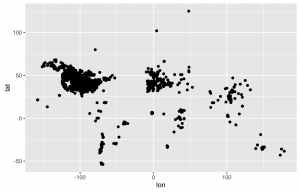Adding some transparency makes it slightly better, but still not satisfactory:

```p + geom_point(alpha=0.25)
```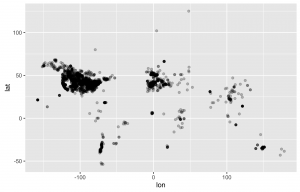Binhex representation makes the things better:

```p + stat_binhex(bins=200, binwidth=c(2,2))
```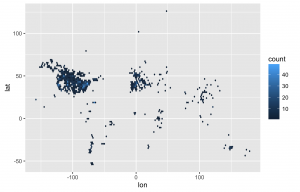It is possible to control the number of bins, and also the bin width. Finally, we can add the land border with geom_path(), tweak the color scale, and make some other minor modifications to the theme:

```p + stat_binhex(bins=200, binwidth=c(2,2)) +
geom_path(data=land,aes(group=group), size=0.25) +